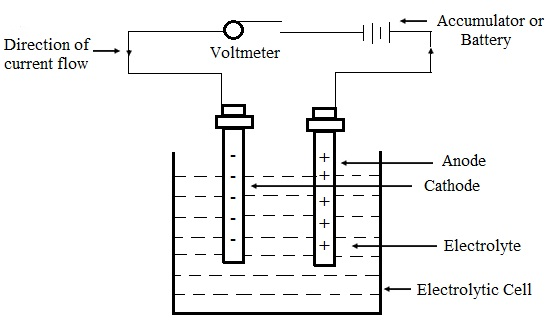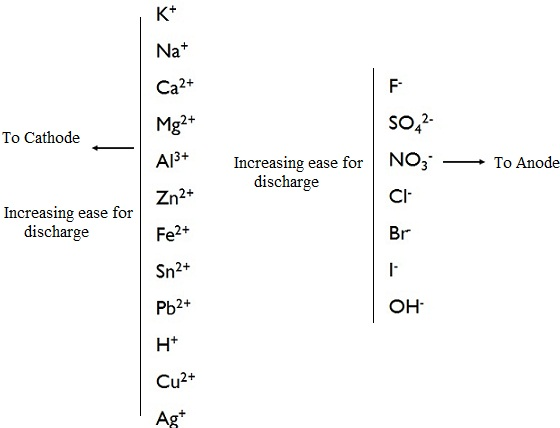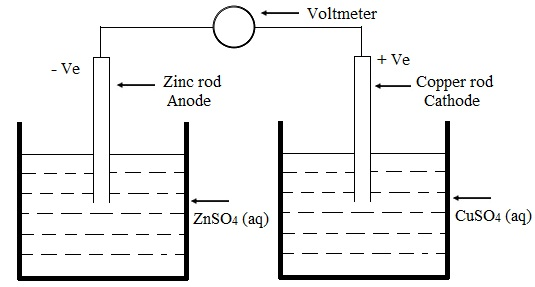#### Electrolysis and Redox Reactions, Chemistry tutorial

Introduction:

Matter is electrical in nature as it includes protons and electrons that carry positive and negative electricity correspondingly. The flow of free electrons comprises an electric current and a steady flow of current supplies electric energy. Most of the material comforts we enjoy nowadays come from the use of current electricity. Electricity plays a huge part in our world that it would be hard to visualize life without it. The working of appliances like radio, computers, television, refrigerators, irons, fans and numerous others is possible as an outcome of electric energy.

Remember the law of conservation of energy that defines that energy can neither be created nor destroyed in any process. This is just changed from one form to the other. Electric energy can be transformed to chemical energy. This is the case if the passage of electricity via matter leads to a chemical reaction. Chemical energy can as well be transformed to electrical energy. This is the case in torch, calculator, car batteries and so on.

Electrolysis:

This is the method through which the passage of an electric current via a solution or molten compound discharges one or more free elements.

Electrolytes and non electrolytes:

Electrolytes are the substances that either in molten or aqueous solution lets the passage of an electric current. Ionic solids comprise of positive and negative ions held altogether in a rigid framework via electrostatic forces. Whenever dissolved in water or melted, the ions become free. Such free ions can carry charge from one point to the other. Illustrations are sodium chloride and calcium chloride. A few covalent compounds, whenever they dissolve in water are dissociated into ions. These will as well conduct electricity. Example: HCl and NH3.

HCl (g) + H2O (l) → H3O+ + Cl-

H3O+ is the hydronium ion. Substances such as NaCl and HCl which for all practical purpose are fully dissociated are termed as strong electrolytes. A few substances such as ethanoic acid (CH3COOH) that dissociates partially in solution are termed as weak electrolytes. Substances which don't conduct electricity either in molten or aqueous solution are known as non-electrolytes. Non-electrolytes are generally covalent or organic compounds. Illustrations are sugar and ethanol.

Electrolytic conduction and electrolysis:Fig: An Electrolytic cell (Voltameter)

The above is an electrolytic cell generally termed as voltameter. Now we shall describe the functions of-the labeled portions of the voltameter. The electrodes are conductors, rods, wires and graphite via that electric current enters and leaves the electrolyte. The anode is the +ve electrode through which current enters the electrolyte. Note that it is joined to the positive terminal of the battery.

The cathode is the negative electrode via which current leaves the electrolyte. This is joined to the negative terminal of the battery. Both the cathode and anode are known as electrodes. Whenever the battery is switched on, the positive ions migrate toward the negative electrode and the negative ions move toward the positive electrode. This movement of ionic charges via the liquid brought about via application of electricity is known as electrolytic conduction.

If the ions of the liquid electrolyte come in contact by the electrodes, chemical reactions take place. At the anode (+ ve electrode), the - ve ions lose electrons. This is known as oxidation.

At cathode (- ve electrode), the + ve ions gain electrons. This is reduction. The liquid will carry on conducting electricity only as long as these reactions at the electrodes continue. The electrons which are deposited at the anode are removed from the solution to maintain a constant supply of electrons at the cathode. As the electrons flow via the exterior wire, ions flow via the solution.

Take for illustration the electrolysis of molten sodium chloride; the ions present are Na+ and Cl-.

At anode,

Cl- → Cl + e

Cl + Cl → Cl2 gas

At cathode, Na+ + e → Na (s)

The total result is that NaCl has been divided into Na solid and Cl2 gas.

Preferential discharge of ions throughout electrolysis:

The product at the electrodes throughout electrolysis based on a number of factors:

1) Nature of the electrode:

Some electrodes take part in electrolytic reactions and should be employed by caution. Platinum and graphite electrodes are inert electrodes and don't take part in the electrolytic reactions. They are safer to employ to avoid unwanted outcomes.

2) Concentration of the ions of the electrolyte:

Rise in the concentration of any ion in the electrolyte raises the chance of its discharge particularly when they are close in the electrochemical sequence. The electrochemical series provides the order of discharge of ions from solution. This factor is significant for mixed electrolyte or for aqueous solutions of electrolytes. Remember that water as well ionizes to give H+ and OH-. Water is a weak electrolyte example: for NaCl (aq)

Cations are Na+ + H3O+

Anions → Cl- OH-

Na+ is too far from H+ on the electrochemical series therefore H+ is discharged as H2(g) at the cathode and Cl2 at the anode.

3) Position on the electrochemical series:Fig: Electrochemical/Reactivity Series

All the other factors being constant, ions which are lower are the series will be discharge in preference to any other above in the series. The electrochemical series is the arrangement of cations and anions in the order they are discharged throughout electrolysis.

Illustrations of electrolysis of some salts:

Let us describe what we have illustrated so far with illustrations: the electrolysis of copper (ii) tetraoxosulphate (iv) and sodium chloride solutions by employing platinum or carbon electrodes. The ions present in sodium chloride solutions are Na+, H3O+ that both migrate to the cathode and Cl- and OH that migrate to the anode. At cathode H3O+ is preferentially discharged and at anode the Cl- is discharged.

H3O+ + e → H2O + H are cathode reactions

H + H → H2 (g)

Cl → Cl + e are anode reactions

Cl + Cl → Cl2 (g)

H2 (g) is given off at cathode and chlorine gas at the anode.

The cations, present in CuSO4 solution are Cu2+, H3O+. Copper is lower than hydrogen in the electrochemical series. This is discharged in preference to the hydrogen.

At anode, the anions are OH- and SO42-. The OH- is much lower in the series than SO42-. This is discharged in preference to the SO42-. The outcome is deposition of copper at the cathode and release of oxygen at the anode.

Cu2+ (aq) + 2e → Cu(s)

4OH- (aq) → O2 (g) + 2H2O (l)

Some more illustrations of electrolysis of salts are represented in the table shown below:

Table: Products at the electrodes throughout the electrolysis of certain salt

 Salt Type of electrode Product at the Cathode Anode Cathode Anode Molten sodium chloride Carbon Carbon Very dilute sodium chloride solution Carbon Carbon H2 (g) O2 (g) Concentrated sodium chloride solution Carbon Carbon H2 (g) Cl2 (g) Concentrated sodium hydroxide solution Platinum or Carbon Platinum or Carbon H2 (g) O2 (g) Dilute sodium hydroxide solution Carbon Carbon H2 (g) O2 (g) Dilute tetraoxosulphate (vi) acid Carbon Carbon H2 (g) O2 (g)

By utilizing the information given in the table, describe why the concentrations of H2SO4 (aq) and NaOH (aq) increase throughout electrolysis of dilute H2SO4 (aq) and NaOH (aq) correspondingly.

Redox Reactions:

Oxidation and reduction:

Oxidation is stated as:

i) Addition of oxygen to a substance.

ii) Elimination of hydrogen from the substance.

iii) Loss of electrons.

iv) Raise in the oxidation number.

Oxidation numbers are numbers provided to elements in the free and combined states according to the set of rules. Oxidation number lets us to keep track of electrons throughout chemical reactions. This is the charge which an atom of the elements would have if both electrons of each and every bond were assigned to the more electronegative element. The elements are assigned zero oxidation numbers in their elemental forms.

Reduction is as well stated as:

i) Elimination of oxygen

iii) Gain of electrons

iv) Reduce in oxidation number

Oxidation and reduction reactions for all time take place together. Remember that during electrolysis oxidation takes place at the anode and reduction at the cathode. The substance which accepts electrons is the oxidizing agent whereas the one which donates the electrons is the reducing agent.

Assignment of oxidation number:

The rules for assigning oxidation to several elements in the compounds are as follows:

1) All atoms of elements in uncombined state encompass zero oxidation number. Example: Na in Na (s), H in H2 (g).

2) In simple ions, example: Na+, Zn2+, Al3+ and so on, the oxidation number is the charge on the ions.

3) The sum of oxidation numbers should add up to zero for compounds and to the total charge on the ions if it is a complex ion.

4) Hydrogen and all alkali metals encompass +1 oxidation number in their compounds. Hydrogen consists of a -1 oxidation number in hydrides

5) The oxidation number of oxygen is -2 in its compound apart from in peroxide where it is -1.

The oxidation number of sulphur in H2SO4 is +6. This is the main reason, why the IUPAC name is tetraoxosulphate (vi) acid.

Redox reactions and the electrochemical cell:

We are familiar that oxidation-reduction reactions take place altogether and they involve loss and gain of electrons correspondingly. This is possible to encompass an arrangement in which the electron made by the oxidation method is made to flow via a conductor (like copper wire) to the reduction site. This flow of electrons will comprise an electric current an illustration of the translation of chemical energy to electrical energy. This kind of arrangement is termed as an electrochemical cell or galvanic cell. An illustration of a galvanic cell is the Daniel cell that makes use of the given Redox reaction.

CuSO4 (aq) + Zn (s) → ZnSO4 (aq) + Cu (s)Fig: Electron transfer in a Redox reaction

For 1.0 mol dm-3 solutions of the electrolytes solutions at 298k the voltmeter will record around 1.10 volts. The reactions that take place are Zn → Zn2+ + 2e- at the anode

Cu2+ + 2e → Cu (s) cathode.

It will be noted that oxidation always take place at the anode and reduction at the cathode. 1.10 volts is known as the electromotive force (emf) of the cell. The emf is the electric potential difference among the electrodes.

Comparison of the electrolytic and electrochemical cells:

The comparison is represented in the table shown below; the differences and similarities are highlighted.

Table: Comparison of the electrolytic and electrochemical cells

 Electrolytic Cell Electrochemical Cell Chemical decomposition takes place through passage of electricity via an electrolyte solution Electricity is produced via chemical reaction in the cell. Electrical energy is transformed to chemical energy Chemical energy is transformed to electrical energy The anode is the positive electrode. The anode is the negative electrode. Oxidation takes place at the anode. Oxidation takes place at the anode. The cathode is the negative electrode. The cathode is the positive electrode. Reduction takes place at the cathode. Reduction takes place at the cathode. Electrons flow from anode to cathode. Electrons flow from anode to cathode

Tutorsglobe: A way to secure high grade in your curriculum (Online Tutoring)